Main Content

# Infinite Array Analysis

This example demonstrates the use of infinite array analysis to model the behavior of a single element - the unit cell embedded in the array - . The array is assumed to be of infinite extent in two dimensions, and located in the XY plane.

### Define Unit Cell

The unit cell refers to a single element in an infinite array. The unit cell element needs a ground plane. Antennas that do not have a groundplane need to be backed by a reflector. A representative example for each case would be dipole backed by a reflector and a microstrip patch antenna. This example will use the dipole backed by a reflector and analyze the unit cell impedance behavior at 10 GHz. The unit cell will has a $\lambda /2$ x $\lambda /2$ cross-section.

```freq = 10e9; vp = physconst('lightspeed'); lambda = vp/freq; ucdx = 0.5*lambda; ucdy = 0.5*lambda;```

Define Individual Element Create a thin dipole of length slightly less than $\lambda /2$ and assign it as the exciter to a reflector of dimensions $\lambda /2$ on each side.

```d = dipole; d.Length = 0.495*lambda; d.Width = lambda/160; d.Tilt = 90; d.TiltAxis = [0 1 0]; r = reflector; r.Exciter = d; r.Spacing = lambda/4; r.GroundPlaneLength = ucdx; r.GroundPlaneWidth = ucdy; figure show(r)```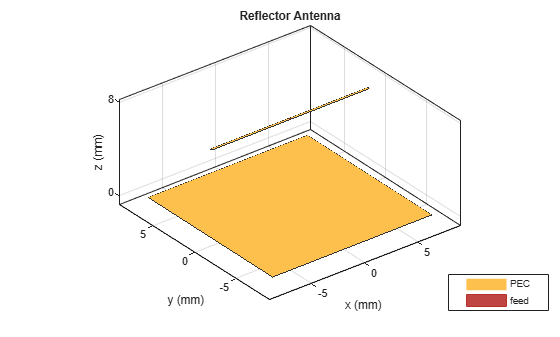### Create Infinite Array

Create the infinite array and assign the reflector backed dipole as the element and view it.

```infArray = infiniteArray; infArray.Element = r; infArrayFigure = figure; show(infArray)```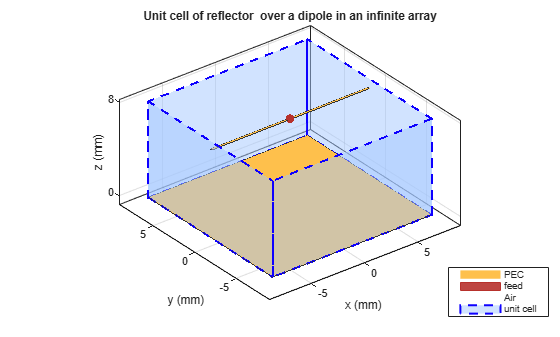### Calculate Scan Impedance

Analyze the impedance behavior of the infinite array by calculating the scan impedance. The scan impedance is the impedance variation of the unit cell element at a single frequency as a function of scan angle. Use the scan angle properties on the infinite array, ScanAzimuth and ScanElevation, to define the scan behavior. Here we calculate the scan impedance in a single plane, defined by azimuth = 0 deg and elevation varying from 0 to 90 deg in 1 deg steps.

```% Scan plane definition az = 0; % E-plane el = 0:1:90; % elevation % Calculate and plot scanZ = nan(1,numel(el)); infArray.ScanAzimuth = az; for i = 1:numel(el) infArray.ScanElevation = el(i); scanZ(i) = impedance(infArray,freq); end figure plot(el,real(scanZ),el,imag(scanZ),'LineWidth',2); grid on legend('Resistance','Reactance') xlabel('Scan Elevation(deg)') ylabel('Impedance (\Omega)') title(['Scan Impedance in az = ' num2str(az) ' deg plane'])```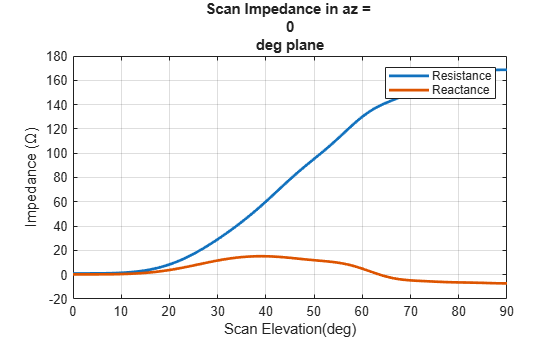### Improving Convergence Behavior

The infinite array analysis depends on a periodic Green's function which comprises of an infinite double summation. For more information about this please refer to the documentation page(`infiniteArray`). The number of terms in this double summation has an impact on the convergence of the results. Increase the number of summation terms to improve the convergence. Executing the command shown will increase the total number of terms to 101 (50 terms each for negative and positive indices, 1 term for 0th term) from the default of 21.

`numSummationTerms(infArray,50);`

The result with higher number of terms is shown in the scan impedance in 3 planes, azimuth = 0, 45 and 90 deg respectively. It takes about 100 seconds per scan plane on a 2.4 GHz machine with 32 GB memory.

```az = [0 45 90]; % E,D,H-plane load scanZData```

E-plane

```figure plot(el,real(scanZ50terms(1,:)),el,imag(scanZ50terms(1,:)),'LineWidth',2); grid on legend('Resistance','Reactance') xlabel('Scan Elevation(deg)') ylabel('Impedance (\Omega)') title(['Scan Impedance in az = ' num2str(az(1)) ' deg plane'])```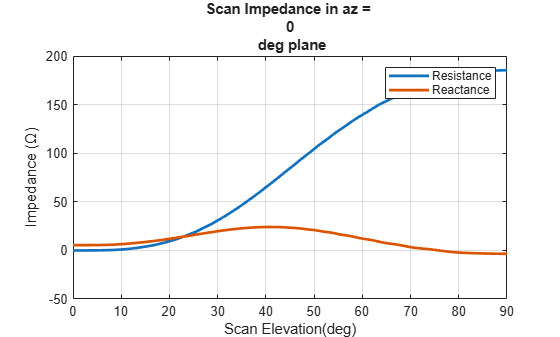D-plane

```figure plot(el,real(scanZ50terms(2,:)),el,imag(scanZ50terms(2,:)),'LineWidth',2); grid on legend('Resistance','Reactance') xlabel('Scan Elevation(deg)') ylabel('Impedance (\Omega)') title(['Scan Impedance in az = ' num2str(az(2)) ' deg plane'])```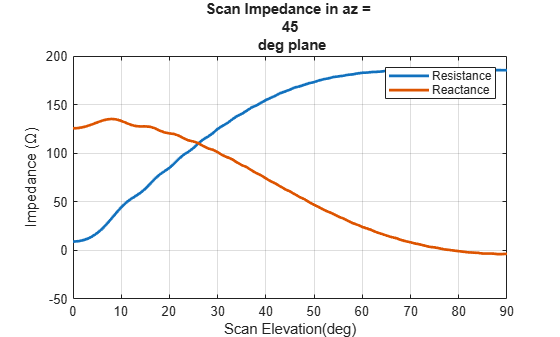H-plane

```figure plot(el,real(scanZ50terms(3,:)),el,imag(scanZ50terms(3,:)),'LineWidth',2); grid on legend('Resistance','Reactance') xlabel('Scan Elevation(deg)') ylabel('Impedance (\Omega)') title(['Scan Impedance in az = ' num2str(az(3)) ' deg plane'])```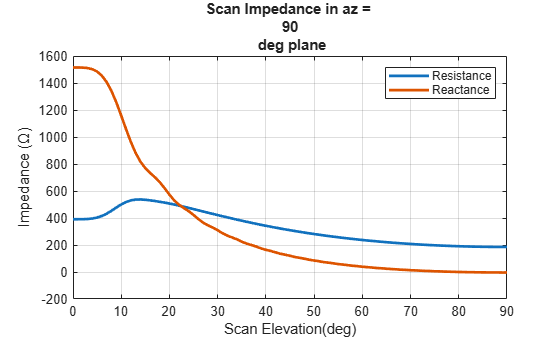### Infinite Array Impedance Variation with Frequency

Fix the scan angle to a specific value and sweep the frequency to observe the impedance behavior of this unit cell element.

```az_scan = 0; el_scan = 45; percent_bw = .15; bw = percent_bw*freq; fmin = freq - bw/2; fmax = freq + bw/2; infArray.ScanAzimuth = az_scan; infArray.ScanElevation = el_scan; figure impedance(infArray,linspace(fmin,fmax,51));```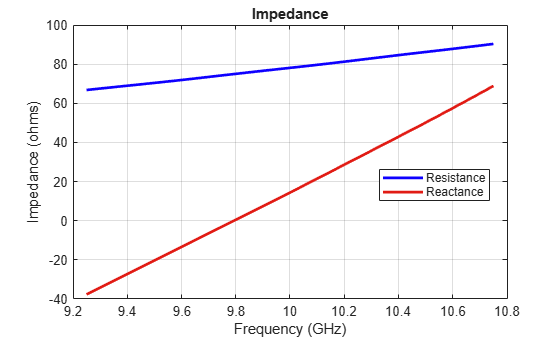### Calculate Isolated Element Pattern and Impedance

Use the scan impedance data from the infinite array analysis to derive the scan element pattern (also known as embedded/array element pattern in case of finite arrays). As indicated in - use the isolated element pattern and impedance to calculate it. Do this by analyzing the dipole backed by an infinite reflector and calculating its power pattern and impedance at 10 GHz.

```r.GroundPlaneLength = inf; r.GroundPlaneWidth = inf; giso = nan(numel(az),numel(el)); gisodB = nan(numel(az),numel(el)); for i = 1:numel(az) giso(i,:) = pattern(r,freq,az(i),el,'Type','power'); gisodB(i,:) = 10*log10(giso(i,:)); gisodB(i,:) = gisodB(i,:) - max(gisodB(i,:)); end Ziso = impedance(r,freq);```

### Calculate and Plot Scan Element Pattern

The calculation of the scan element pattern requires that we define the generator impedance. Here we choose it to be the broadside scan resistance.

```Rg = 185; Xg = 0; Zg = Rg + 1i*Xg; gs = nan(numel(az),numel(el)); gsdB = nan(numel(az),numel(el)); for i = 1:numel(az) gs(i,:) = 4*Rg*real(Ziso).*giso(i,:)./(abs(scanZ50terms(i,:) + Zg)).^2; gsdB(i,:)= 10*log10(gs(i,:)); gsdB(i,:)= gsdB(i,:) - max(gsdB(i,:)); end figure; plot(el,gsdB(1,:),el,gsdB(2,:),el,gsdB(3,:),'LineWidth',2.0) grid on axis([0 90 -20 0]) xlabel('Scan Elevation (deg.)') ylabel('Power pattern(dB)') title(strcat('E-Plane (az = 0 deg.) Power Pattern')) legend('az = 0 deg','az = 45 deg','az = 90 deg','Location','best')```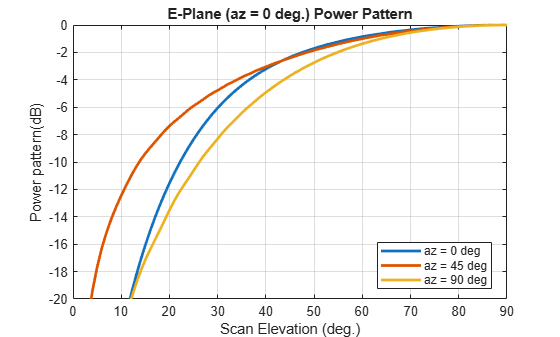### Reference

 J. Allen, "Gain and impedance variation in scanned dipole arrays," IRE Transactions on Antennas and Propagation, vol.10, no.5, pp.566-572, September 1962.

 R. C. Hansen, Phased Array Antennas, Chapter 7 and 8, John Wiley & Sons Inc.,2nd Edition, 1998.

 R. J. Mailloux, 'Phased Array Antenna Handbook', Artech House,2nd edition, 2005

 W. Stutzman, G. Thiele, 'Antenna Theory and Design', John Wiley & Sons Inc., 3rd Edition, 2013.

## Support

#### Hybrid Beamforming for Massive MIMO Phased Array Systems

Download the white paper